Practice Test: Civil Engineering (CE)- 15

# Practice Test: Civil Engineering (CE)- 15

Test Description

## 65 Questions MCQ Test | Practice Test: Civil Engineering (CE)- 15

Practice Test: Civil Engineering (CE)- 15 for Civil Engineering (CE) 2023 is part of Civil Engineering (CE) preparation. The Practice Test: Civil Engineering (CE)- 15 questions and answers have been prepared according to the Civil Engineering (CE) exam syllabus.The Practice Test: Civil Engineering (CE)- 15 MCQs are made for Civil Engineering (CE) 2023 Exam. Find important definitions, questions, notes, meanings, examples, exercises, MCQs and online tests for Practice Test: Civil Engineering (CE)- 15 below.
Solutions of Practice Test: Civil Engineering (CE)- 15 questions in English are available as part of our course for Civil Engineering (CE) & Practice Test: Civil Engineering (CE)- 15 solutions in Hindi for Civil Engineering (CE) course. Download more important topics, notes, lectures and mock test series for Civil Engineering (CE) Exam by signing up for free. Attempt Practice Test: Civil Engineering (CE)- 15 | 65 questions in 180 minutes | Mock test for Civil Engineering (CE) preparation | Free important questions MCQ to study for Civil Engineering (CE) Exam | Download free PDF with solutions
 1 Crore+ students have signed up on EduRev. Have you?
Practice Test: Civil Engineering (CE)- 15 - Question 1

### For a > b, if a + b = 5 and ab = 6, then the value of (a2 - b2) is

Detailed Solution for Practice Test: Civil Engineering (CE)- 15 - Question 1 Since, (a + b)2 = a2 + b2 + 2ab and (a – b)2 = a2 + b2 - 2ab

→ (a - b)2 = (a + b)2– 4ab ………………….(equation)

Given, (a + b) = 5 and ab = 6

Substituting the values in equation, we get,

(a-b)2 = 52 – 4 x 6 = 1

→ (a - b) = 1

We know that, (a2 – b2) = (a + b)(a - b)

Substituting the values of (a + b) and (a - b) in above equation, we get,

(a2 – b2) = 5 × 1 = 5

Hence, the required answer is 5.

Another Solutions:

let, a= 3 & b=2 a>b

a+b=5

3+2= 5,

ab =6, 2*3=6,

(a2 - b2)= 32- 22 = 9- 4 = 5.

Practice Test: Civil Engineering (CE)- 15 - Question 2

### In the following question, out of the given alternatives, select the word opposite in meaning to the given word.Inevitable

Detailed Solution for Practice Test: Civil Engineering (CE)- 15 - Question 2 Inevitable (adj.): certain to happen, unavoidable.

Obligatory (adj.): so customary or fashionable as to be expected of everyone or on every occasion.

Prescribed (adj.): state authoritatively or as a rule that (an action or procedure) should be carried out.

Avoidable (adj.): able to be avoided or prevented.

So, the correct answer is option D.

Practice Test: Civil Engineering (CE)- 15 - Question 3

### What is the difference between the expenditure made by a university for the publication of journals and psychology?Total Expenditure : Rs. 60 LacsExpenditure of funds By University for various purposes

Detailed Solution for Practice Test: Civil Engineering (CE)- 15 - Question 3 Expenditure made by university of publication of journals

= 15% of 60 lacs

= 15 × 60 lacs/ 100

= 9 lacs

Expenditure made by university of psychology

= 10% of 60 lacs

= 10 × 60 lacs/100

= 6 lacs

Difference between the expenditure made by university of publication of journals and psychology

= 9-6

= 3 lacs

Practice Test: Civil Engineering (CE)- 15 - Question 4

In the following questions groups of four words are given. In each group, one word is spelt.

Find the correctly spelled word.

Detailed Solution for Practice Test: Civil Engineering (CE)- 15 - Question 4 The correctly spelled word is ‘Propitiate’ that means proliferate, appropriate, appreciate.
Practice Test: Civil Engineering (CE)- 15 - Question 5

Select the most appropriate antonym of the given word.

INNOCUOUS

Detailed Solution for Practice Test: Civil Engineering (CE)- 15 - Question 5 Let us understand the meaning of the given words :-

Innocuous = not harmful or offensive.

Eg. : The conversation was innocuous and she wasn't identified by name.

Loquacious = tending to talk a great deal; talkative.

Deadly = causing or able to cause death.

Eg. : He was acquitted on charges of assault with a deadly weapon.

Ferocious = frightening and violent.

Harmless = not causing or capable of causing harm.

Hence, option C is the correct answer.

Practice Test: Civil Engineering (CE)- 15 - Question 6

Choose the most appropriate word from the options given below to complete the following sentence.

Communication and interpersonal skills are ......... important in their own ways.

Detailed Solution for Practice Test: Civil Engineering (CE)- 15 - Question 6

The correct word is “both” as communication and interpersonal skills are two skills that are important.

Practice Test: Civil Engineering (CE)- 15 - Question 7

In the following question, some of the sentences have errors and some have none. Find out which part of a sentence has an error. The number of that part is the answer. If there is no error, your answer is (4) i.e., No error.

We requested the watchman (1)/ to clean up the basement (2)/ so that the children had enough space to play (3)/ No error (4)

Detailed Solution for Practice Test: Civil Engineering (CE)- 15 - Question 7

We ordered/asked the watchman should be used as the sense of the given sentence shows order. Hence, there is an error in (1).

Practice Test: Civil Engineering (CE)- 15 - Question 8

The Palghat Gap (or Palakkad Gap), a region about 30 km wide in the southern part of the Western Ghats in India, is lower than the hilly terrain to its north and south. The exact reasons for the formation of this gap are not clear. It results in the neighbouring regions of Tamil Nadu getting more rainfall from the South West monsoon and the neighbouring regions of Kerala having higher summer temperatures.

Q. What can be inferred from this passage?

Detailed Solution for Practice Test: Civil Engineering (CE)- 15 - Question 8

Here the passage is asking, ” what can be inferred ? “, i.e. it’s asking about the hidden conclusion of the passage.

Why not Option A ? – because it is clearly given in the passage : “The exact reasons for the formation of this gap are not clear “.

Why not Option B ? – because the passage nowhere talks about the low-lying regions of Kerala and Tamil Nadu near the Palghat Gap.

Why not option D ? – Because the passage only says : “neighbouring regions of Kerala having higher summer temperatures”, it doesn’t say that this high temperature results in rainfall.

Note: We have to think and conclude only from what is given in the passage. We can’t make our own conclusions.

Why Option C ? – because the passage says : ” It results in the neighbouring regions of Tamil Nadu getting more rainfall from the South West monsoon and the neighbouring regions of Kerala having higher summer temperatures”,

Hence we can conclude here that the weather is getting affected due to the Gap.

Therefore, option C is correct.

Practice Test: Civil Engineering (CE)- 15 - Question 9

It takes 30 minutes to empty a half-full tank by draining it at a constant rate. It is decided to simultaneously pump water into the half-full tank while draining it. What is the rate at which water has to be pumped in so that it gets hilly filled in 10 minutes?

Detailed Solution for Practice Test: Civil Engineering (CE)- 15 - Question 9

Given in the question that; Vhalf = 30(s)

Let us take drawing rate = s

Total volume = 60 S tank

(s1)(10) – (s)10 = 30s

s1(s) – s = 3s

s1 = 4s

s1 = 4 times the drawing rate.

Practice Test: Civil Engineering (CE)- 15 - Question 10

While receiving the award, the scientist said, "I feel vindicated”. Which of the following is closest in meaning to the word ‘vindicated’?

Detailed Solution for Practice Test: Civil Engineering (CE)- 15 - Question 10

Vindicated means freed from any question of guilt, so “substantiated” is the closest.

Practice Test: Civil Engineering (CE)- 15 - Question 11

The area enclosed between the parabola y = x2 and the straight line y = x is

Detailed Solution for Practice Test: Civil Engineering (CE)- 15 - Question 11 Given: y = x2 & y = x.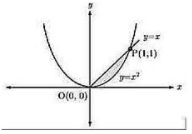On solving given equation, we get the intersection points as,

y = x2 puty = x

x = x2

x2−x = 0

x(x−1) = x = 0,1

Then from y = x

For x = 0 y = 0

& x = 1 y = 1

We can see that curve y = x2 and y = x intersects at point (0,0) and (1,1) So, the area bounded by both the curves is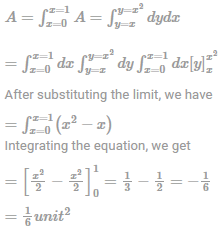Area is never Negative.

Practice Test: Civil Engineering (CE)- 15 - Question 12

Which of the following statement is INCORRECT?

Detailed Solution for Practice Test: Civil Engineering (CE)- 15 - Question 12
• Shear parameters ‘c’ and ∅ are dependent of water content of soil

• Vane shear test is a useful method of measuring the shear strength of clay. It is a cheaper and quicker method. The test can also be conducted in the laboratory.

• The laboratory vane shear test for the measurement of shear strength of cohesive soils, is useful for soils of low shear strength (less than 0.3kg/{cm}^2) for which triaxial or unconfined tests cannot be performed.

• The test gives the undrained strength of the soil. The undisturbed and remoulded strength obtained are useful for evaluating the sensitivity of soil.

This test is also useful when the soil is soft and its water content is nearer to the liquid limit.

Practice Test: Civil Engineering (CE)- 15 - Question 13

A manometer is used to measure the pressure in a tank. The fluid used has a specific gravity of 0.86, and the manometer column height is 50cm, as shown in figure If the local atmospheric pressure is 100kPa, calculate the absolute pressure within the tank.

Take the standard density of water to be 1000kg/m3.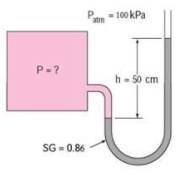Detailed Solution for Practice Test: Civil Engineering (CE)- 15 - Question 13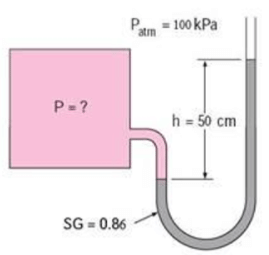The specific gravity of the manometer fluid is given to be 0.86

ρ = SG(ρH2O) = (0.86) (1000 kg/m3) =860 kg/m3

Then from Eq. ,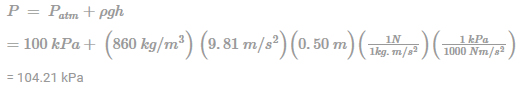Practice Test: Civil Engineering (CE)- 15 - Question 14

A construction project consists of twelve activities. The estimated duration (in days) required to complete each of the activities along with the corresponding network diagram is shown below.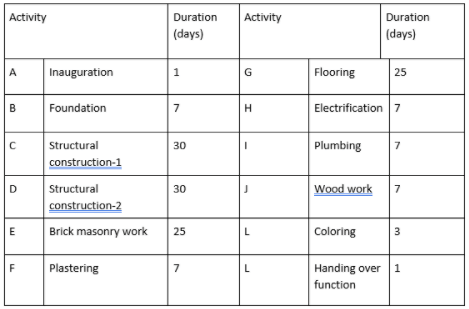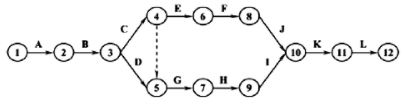Total floats (in days) for the activities 5-7 and 11-12 for the project are, respectively,

Detailed Solution for Practice Test: Civil Engineering (CE)- 15 - Question 14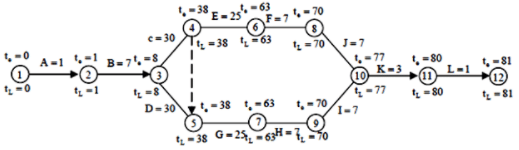(5)-(7) = Total float = te-tL= 0

(11)-(12) = Total Float = te-tL = 0

Practice Test: Civil Engineering (CE)- 15 - Question 15

A cylindrical shell 1000 mm long, 150 mm internal diameter, having a thickness of 8 mm is filled with a fluid at atmospheric pressure. An additional 25000 mm3 of fluid is pumped into the cylinder, then the hoop stress-induced will be ___________ N/mm2[E=2×105 N/mm2 and μ=0.3]

Detailed Solution for Practice Test: Civil Engineering (CE)- 15 - Question 15 Volume tics train=Change in volume/Original volume=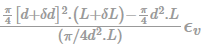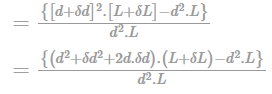simplifying and neglecting the products and squares of small quantities,

i.e .δdδL , hence = 2d.δd.L+δL.d2/d2L

= δL/L+ 2.δd/d Bt defintion δL/L

= Longitudinal strain

δdd= hoop strain

Thus Volumetric strain = longitudinal strain + 2 × hoop strain

ϵV = 2ϵh + ϵL

25000/π/4(150)2 × 1000

= pD/4tE(5-4μ) ⇒ 1.4147×10-3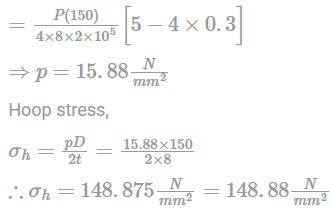Practice Test: Civil Engineering (CE)- 15 - Question 16

There is a free overfall at the end of a long open channel. For a given flow rate, the critical depth is less than the normal depth. What gradually varied flow profile will occur in the channel for this flow rate?

Detailed Solution for Practice Test: Civil Engineering (CE)- 15 - Question 16 For a given flow rate, if critical depth (Yc) is less than normal depth (Yn)

i.e. if Yc < Yn

Then, the flow profile will be M2.

Practice Test: Civil Engineering (CE)- 15 - Question 17

For a given PERT activity, the standard deviation of the time needed to complete an activity is 3. If the optimistic time and the most likely time required to complete this activity are 8 and 12 minutes respectively. Find out the expected time in order to complete this activity.

Detailed Solution for Practice Test: Civil Engineering (CE)- 15 - Question 17 Reason: σ = tp - t0/6

σ = 3 = (tp-t0)/6

tp-t0 = 6×3, tp-t0 = 18

We know that, t0 = 8

So,

tp- 8 =18 And, therefore, tp = 26

We have, te = t0+4tm+tp/6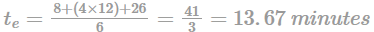Practice Test: Civil Engineering (CE)- 15 - Question 18

The concentration of Sulfur Dioxide (SO2) in the ambient atmosphere was measured as 30 µg/m3. Under the same conditions, the above SO2 concentration expressed in ppm is ___________.

Given: (P/(RT) = 41.6 mol/m3; where, P = Pressure; T = Temperature; R – universal gas constant; Molecular weight of SO2 = 64.

Detailed Solution for Practice Test: Civil Engineering (CE)- 15 - Question 18 1 m3 of air has 30 mg SO2

106 m3 of air has 30g SO2

= 30/64molSO2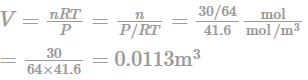Concentration of SO2 in ppm = 0.0113 ppm

Practice Test: Civil Engineering (CE)- 15 - Question 19

A steady, incompressible flow is given by:

u = 2x2 + y2 and v= -4xy , What is the convective acceleration along x-direction at point (1, 2)

Detailed Solution for Practice Test: Civil Engineering (CE)- 15 - Question 19 Convective acceleration along x direction at point (1,2)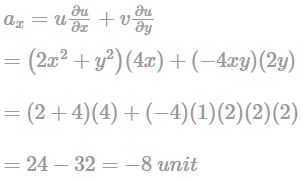Practice Test: Civil Engineering (CE)- 15 - Question 20

A two-faced fair coin has its faces designated as head (H) and tail (T). This coin is tossed three times in succession to record the following outcomes: H, H, H. If the coin is tossed one more time, the probability (up to one decimal place) of obtaining H again, given the previous realizations of H, H and H, would be_____.

Detailed Solution for Practice Test: Civil Engineering (CE)- 15 - Question 20 Given the first three are already heads. If the coin is tossed again, the outcome does not depend on previous outcomes.

Probability getting head = 1/2 = 0.5.

Practice Test: Civil Engineering (CE)- 15 - Question 21

The function f(x) = 2x3 – 3x2 – 36x + 2 has its maxima at

Detailed Solution for Practice Test: Civil Engineering (CE)- 15 - Question 21 For maximum f(x),

⇒ f'(x) = 0

⇒ 6x2−6x−36=0

⇒ x2−x−6=0

⇒ x2−3x+2x−6=0

⇒ x(x-3)+2(x-3)=0

⇒ x = 3, -2

∴ f''(x) = 12x-6

⇒ f''(x)<0 @x=-2

So, f(x) has a maximum value at -2 only.

Practice Test: Civil Engineering (CE)- 15 - Question 22

The ratio of tension and shear force in each bolt of the joint, as shown in figure, is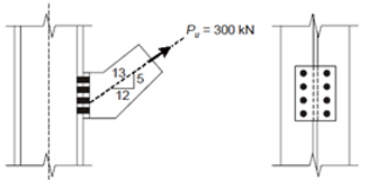Detailed Solution for Practice Test: Civil Engineering (CE)- 15 - Question 22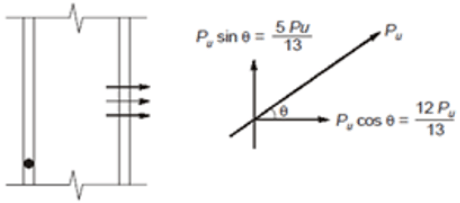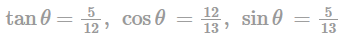Tension in each bolt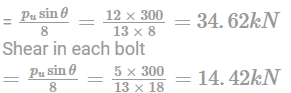The ratio of tension and shear in each bolt

= Tension in each bolt / Shear in each bolt

= 34.62/14.42 = 2.40

Practice Test: Civil Engineering (CE)- 15 - Question 23

Three values of x and y are to be fitted in a straight line in the form y = a + bx by the method of least squares. Given : N=3, ∑x = 6, ∑y = 21, ∑x2 = 14 and ∑xy = 46, the values of a and b are respectively.

Detailed Solution for Practice Test: Civil Engineering (CE)- 15 - Question 23 Given,

N = 3, ∑x = 6, ∑y = 21, ∑x2 = 14 and ∑xy = 46

There are two normal equations to evaluate a and b of the equation

y = a + bx

∑y = Na + b∑x …(i)

and ∑xy = a∑x + b∑x2 …(ii)

From Eq. (i), 21 = 3a + 6b …(iii)

From Eq. (ii) 46 = 6a + 14b

⇒ 23 = 3a + 7b …(iv)

Now, Eq. (iv) – Eq. (iii),

3a + 7b = 23

3a + 6b = 21

- - -

b = 2

From Eq. (iii), 3a = 21 – 12

3a = 9 ⇒ a = 3

Practice Test: Civil Engineering (CE)- 15 - Question 24

A RC column of square cross-section (400×400mm2) has its column load-moment interaction diagram as shown in figure below.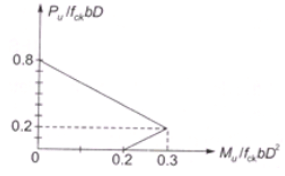Detailed Solution for Practice Test: Civil Engineering (CE)- 15 - Question 24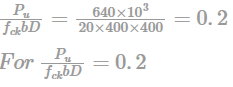We have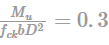from the interaction diagram ∴Mu 0.3 x 20 x 400 x 4002 x 10-3 ⇒ Mu = 384000 kN -mm

e = Mu/Pu = 384000/640 = 600 mm

Practice Test: Civil Engineering (CE)- 15 - Question 25

At two points 1 and 2 in a pipeline the velocities are V and 2V, respectively. Both the points are at the same elevation. Both the points are at the same elevation. The fluid density is ρ. The flow can be assumed to be compressible, inviscid, steady and irrotational. The difference in pressures P1 and P2 at points I and 2 is

Detailed Solution for Practice Test: Civil Engineering (CE)- 15 - Question 25 Applying Bernouli's theorem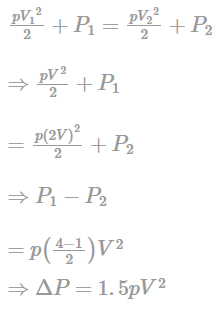Practice Test: Civil Engineering (CE)- 15 - Question 26

In a network, a project activity has normal cost and normal duration of Rs. 400 and 8 days respectively. The same activity has crash cost and crash duration of Rs. 800 and 6 days. The crash cost slope of activity is;

Detailed Solution for Practice Test: Civil Engineering (CE)- 15 - Question 26 Cost Slope: In actual practice, we assume the time-cost curve to be linear between crash point A and Normal point B. The curve(shown below) shows that there is a change in cost for change in time. The term ‘cost-slope’ is defined as the “increase in the cost of the activity per unit decrease in the time”.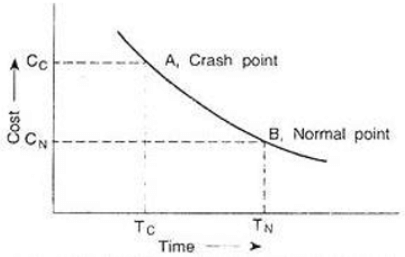Mathematically, we can say,

Cost slope = [Crash cost – Normal cost]/[Normal time – Crash time]

In simple words, we can say that the cost slope of an activity is the increase in cost of an activity by reducing the duration by one unit.

So, Cost Slope = (Crash Cost-Normal Cost)/(Normal time - Crash time) = 800-400/8-6 = 200.

Practice Test: Civil Engineering (CE)- 15 - Question 27

Precast concrete pile of size 50 cm × 50 cm is to be driven into clay strata. The unconfined compressive strength of which is 110 kN/m2. Then the length required to carry a safe working load of 500 kN with FOS = 3 and α = 0.6 is _______ m.

Detailed Solution for Practice Test: Civil Engineering (CE)- 15 - Question 27 Soil is clay ≡ point bearing

Qu = CubNcAb + aCu avAs

Qu = 500 × FOS = 500×3 = 1500

1500 = 110/2 × 9 × 0.52 + 0.6 × 110/2 × 4 × 0.5 × L

L = 20.8522 m.

Practice Test: Civil Engineering (CE)- 15 - Question 28

An RC beam of rectangular cross-section has 250 mm width and 450 mm effective depth. Vertical stirrups 2-legged 10 mm diameter are provided at a spacing of 250 mm centre to centre and assume design shear strength of concrete is 0.6 MPa. The total shear capacity (in kN) of the section is

[Use Fe 415 grade steel for stirrups]

Detailed Solution for Practice Test: Civil Engineering (CE)- 15 - Question 28 Shear resistance of shear reinforcement,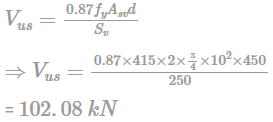Shear resistance of concrete,

Vus = τcbd = 0.6 × 250 × 450 = 67.5kN

∴ Total shear capacity = Vus + Vus = 169.58kn

Practice Test: Civil Engineering (CE)- 15 - Question 29

The command area of a channel is 4000 hectares. The intensity of irrigation of a crop is 70% and it requires 60 cm of water in 15 days, when the effective rainfall is recorded as 15 cm during that period, then the duty at the head of the channel is _________ ha/cumec.

[Assume total losses as 15%]

Detailed Solution for Practice Test: Civil Engineering (CE)- 15 - Question 29

Depth of water = 60cm

Effective rainfall = 15 cm

Depth of irrigating water = 60 – 15 = 45 cm

⇒ Delta = 45 cm = 0.45 m

B = 15 days

From relation, ∆= 8.64×B/D

⇒ Duty(D) = 8.64×15/0.45 = 288ha/cumec

Due to loss of water, duty at head of channel is reduced

Here losses are 15%

So, duty at head of channel = 288 × 85/100

= 244.80 ha/cumecs

Practice Test: Civil Engineering (CE)- 15 - Question 30

A horizontal water jet with a velocity of 10 m/s and cross-sectional area of 10mm2 strikes a flat plate held normal to the flow direction. The density of water is 1000kg/m3. The total force on the plate due to the jet is

Detailed Solution for Practice Test: Civil Engineering (CE)- 15 - Question 30 Density of water (ρ) = 1000 kg/m3

Velocity of water (υ) =10 m/s

Cross section of jet, A = 10 mm2

Force on Plate due to jet of water F = ρυ2A

= 1000 kg/m3×(10 m/sec)2 × 10 × 10-6 m2

= 1 N

Practice Test: Civil Engineering (CE)- 15 - Question 31

A man, 65 kg, descends to the ground with the help of a parachute, 18 kg. The parachute is hemispherical in shape, 2m diameter. Density of air can be taken as 0.00125 g/cm3 and its kinematic viscosity as 0.15 stoke. What is the terminal velocity of the parachute?

(Take CD = 1.5 and g = 1000 cm/sec2)

Detailed Solution for Practice Test: Civil Engineering (CE)- 15 - Question 31 For terminal velocity total weight of man and parachute will be balanced by drag force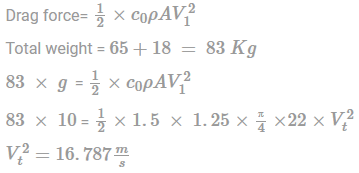So, terminal velocity = 16.78 m/s

Practice Test: Civil Engineering (CE)- 15 - Question 32

The eigenvalue of a symmetric matrix are all;

Detailed Solution for Practice Test: Civil Engineering (CE)- 15 - Question 32 Consider a symmetric matrix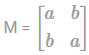The characteristic equation for this matrix is |M-λI| = 0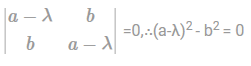a - λ = ±b

∴ λ = a±b is a real value

Therefore, for a real symmetric matrix eigen values are real.

Practice Test: Civil Engineering (CE)- 15 - Question 33

Assuming that the river bed level does not change and the depth of water in the river was 10m, 15m and 8m during the months of February, July and December respectively of a particular year. The average bulk density of the soil is 20kN/mJ3. The density of water is 10kN/m3. The effective stress at a depth of 10m below the river bed during these months would be

Detailed Solution for Practice Test: Civil Engineering (CE)- 15 - Question 33 Since, effective stress at any depth does not depends upon variation of water level above the bed of river,

∴ σ¯= σ − u = γh − γwh

= 20×10−10×10=100kN/m2

Hence, 100kN/m2 in Feb, 100kN/m2 in July and 100kN/m2 in December

Practice Test: Civil Engineering (CE)- 15 - Question 34

Find the horizontal thrust for the frame at A.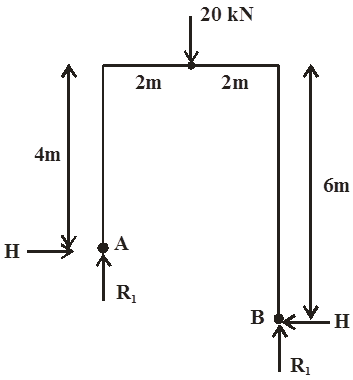Detailed Solution for Practice Test: Civil Engineering (CE)- 15 - Question 34

H(2) + R1(4) = 20*2

H + 2R1 = 20

H(4) = R1 * 2

R1 = 2H

Therefore H = -4KN

Practice Test: Civil Engineering (CE)- 15 - Question 35

For a two dimensional flow field, the stream function Y is given as Ψ = 3/2(y2−x2). The magnitude of discharge occurring between the streamlines passing through points (0, 3) and (3, 4) is:

Detailed Solution for Practice Test: Civil Engineering (CE)- 15 - Question 35 Given, Ψ = 3/2(y2−x2) and points (0, 3) and (3, 4)

So, Q = ψ1 − ψ2

= 5/2(9−0) − 3/2(16−9)

= 3 units.

Practice Test: Civil Engineering (CE)- 15 - Question 36

A piece of material is subjected to two perpendicular stresses 112 N/mm2 (tensile) and p (compressive). If the strain energy due to both the stresses is same as a stress of 128 N/mm2 acting alone, then the compressive stress, p (in N/mm2), is;

[Take Poisson’s ratio (μ)= 0.3]

Detailed Solution for Practice Test: Civil Engineering (CE)- 15 - Question 36

As we know the relation,

Strain energy per unit volume,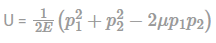Here, P1 = 112 N/mm2 (tensile)

P2 = p N/mm2 (compressive)

μ = 0.3

∴ U = 1/2E(1122 + p2 + 2 × 0.3 × 112p

U = 1/2E (12544 + 67.2p + p2) ...(i)

For a single stress, p’, acting alone , U = p1/2/2E per unit volume (wehere, p’ = 128 N/mm2)

⇒ 1282/2E = 1/2E(12544 + 67.2p + p2)

⇒ p2 + 67.2p - 3840 = 0

∴ p = 36.89 N/ mm2

Practice Test: Civil Engineering (CE)- 15 - Question 37

A pre-tensioned concrete member if section 200×250 mm contains tendons of area 500 mm2 at centre of gravity of the section. The pre-stress in the tendons is 1000N/mm2. Assuming modular ratio as 10.the stress in(N/mm2) concrete is

Detailed Solution for Practice Test: Civil Engineering (CE)- 15 - Question 37

Compressive force in steel = Ast × fst

= 500 × 103 = 5 × 105

Area of section in terms of concrete = A +(m-1) Ast

= (200×250)+(10-1) × 500

= 54500 mm2

Stress in concrete = PA = 5 × 105/54500 = 9.17 N/mm2

Practice Test: Civil Engineering (CE)- 15 - Question 38

Consider the following statements regarding two hinged arch.

1) Two hinged arch is more stable than three hinged arches and it is more generally used in structures inspite of its indeterminacy.

2) The horizontal reaction at the two hinged arch should be kept minimum for the maximum utilization of the arch.

Which of the above statements are incorrect?

Detailed Solution for Practice Test: Civil Engineering (CE)- 15 - Question 38

A two hinged arch should produce the horizontal reaction because the horizontal reaction reduces the bending moment of the arch as compared to the simply supported beam, thus it should not be minimized. So, option B is incorrect.

Practice Test: Civil Engineering (CE)- 15 - Question 39

A rectangular foundation of size 4m x 3m exerts a pressure of 25 kN/m2. The vertical stress at a point X located below 3m as shown in the figure is;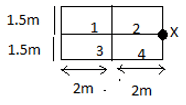Detailed Solution for Practice Test: Civil Engineering (CE)- 15 - Question 39

Let the total loaded area be divided into 4 small areas of size 2m x 1.5m.

Load on each area = 25 x (2x1.5) = 75 kN

The stress at point X is determined due to 4 point loads, using Boussinesq’s equation.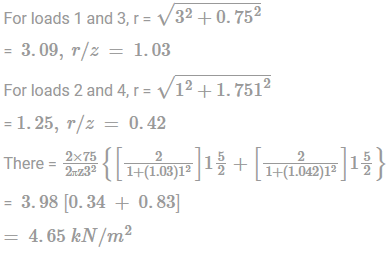Practice Test: Civil Engineering (CE)- 15 - Question 40

A cable of length 20m is supported at two ends at same level as shown in figure. The Horizontal distance between the supports is 16m. A pt. load of 100 kN is applied at pt. R which divides it into equal parts.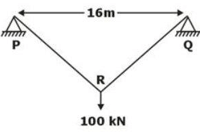Neglecting the self wt. of the cable, the tension (in kN, integer value) in the cable due to applied load will be __________.

Detailed Solution for Practice Test: Civil Engineering (CE)- 15 - Question 40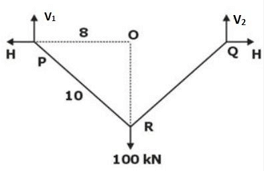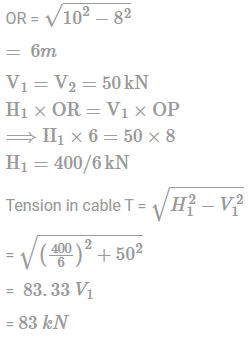*Answer can only contain numeric values
Practice Test: Civil Engineering (CE)- 15 - Question 41

A Cipolletti weir discharges water with the head of water over the crest being 20 cm. If the head due to velocity of approach is 0.02 m, what will be the excess percentage of flow, when corrected for velocity of approach as compared to when not corrected?

Detailed Solution for Practice Test: Civil Engineering (CE)- 15 - Question 41 When velocity of approach is taken into consideration,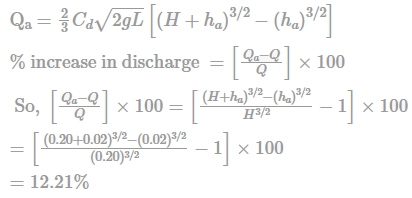*Answer can only contain numeric values
Practice Test: Civil Engineering (CE)- 15 - Question 42

In the gulp method of stream gauging by dilution technique, 50 liter of chemical X with concentration of 240g/L is introduced suddenly into the stream at a section. At a downstream monitoring section the concentration profile of chemical X that crossed the section was found to be a triangle with a base of 10 hours and a peak of 0.01 ppm. Estimate the discharge in stream in cumec. (Up to 1 decimal place)

Detailed Solution for Practice Test: Civil Engineering (CE)- 15 - Question 42 Area of time concentration curve at d/s section = 1/2 = {2}\)×10×3600×0.01×10-6= 180×10-6

The weight of tracer introduced in gulp = 50 × 240g = 12 kg = 117.72N

Taking the unit weight of water as 9810N/m3

180×10-6 × 9810 Q = 117.72

Q = 66.667 m3/s.

Practice Test: Civil Engineering (CE)- 15 - Question 43

What will be the FEM at point A in beam AB?

Detailed Solution for Practice Test: Civil Engineering (CE)- 15 - Question 43 Since there is no external load acting on beam AB, there won’t be any FEM at point A. Hence, the correct answer is 0.
Practice Test: Civil Engineering (CE)- 15 - Question 44

The beam shown in the given figure has a design bending moment of.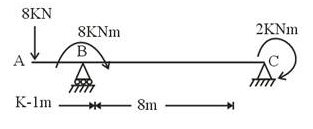Detailed Solution for Practice Test: Civil Engineering (CE)- 15 - Question 44

∑M@C=0

8 × 9 − 8 − RB × 8 − 2 = 0

RB = 72−10/8 = 7.75

RC = 8 - 7.75 = 0.25

BMA = 0

BMB = 8×1 = 8 KNm (just right to B)

BMB = 8×1 – 8 = 0 (Just left to B)

BMC = 2 KNm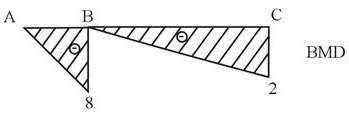Design BM = 8K Nm

Practice Test: Civil Engineering (CE)- 15 - Question 45

Consider the following activity of a certain network: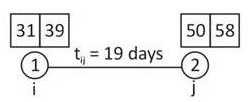The value of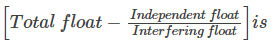Detailed Solution for Practice Test: Civil Engineering (CE)- 15 - Question 45

Total float = (TL)j — (TE)i — tij

Total float = 58 — 31 — 19 = 8 days

Free float = (TE)j — (TE)i — Tij

Free float = 50 — 31 — 19 = 0

Independent float = (TE)j — (TL)i — tij

Independent float = 50 — 39 — 19 = — 8

Interfering float = total float — free float

Interfering float = 8 — 0 = 8 days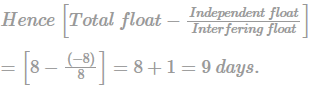*Answer can only contain numeric values
Practice Test: Civil Engineering (CE)- 15 - Question 46

Consider a 6m concrete beam of rectangular cross-section having an effective length of 9 m and subjected to load 15 kN/m. The section size is 400 mm×800 mm. Use M30 concrete and Fe500.

The maximum bending moment occuring in the beam is _____ kNm.

Detailed Solution for Practice Test: Civil Engineering (CE)- 15 - Question 46 Provided d = 800 mm, b = 400 mm

= (0.8×0.4×1×25) + 15

= 23 kN/m

Factored Load = 23×1.5 = 34.5 kN/m

Bending Moment =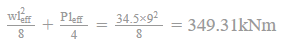Practice Test: Civil Engineering (CE)- 15 - Question 47

A project network is shown below. Numbering according to Fulkerson’s rule the node numbers corresponding to events A, B, C, D, E, F, G, H and I are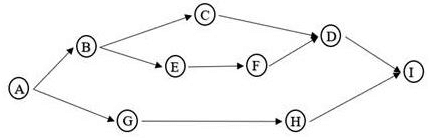Detailed Solution for Practice Test: Civil Engineering (CE)- 15 - Question 47

The numbering should be done such that the logical sequence is maintained.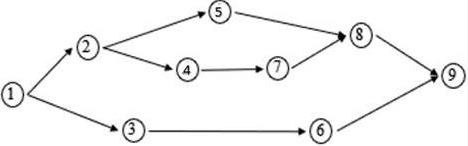Hence, the corresponding node numbers are 1, 2, 5, 8, 4, 7, 3, 6 and 9.

Practice Test: Civil Engineering (CE)- 15 - Question 48

Consider the following network diagram, where σ is the standard deviation for a particular activity (under the normal distribution curve, the probability at Z = 1 is 84.13%).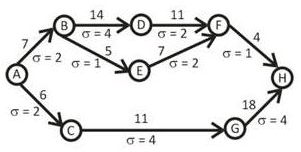The probability of completing the project before 31 days is __________%.

Detailed Solution for Practice Test: Civil Engineering (CE)- 15 - Question 48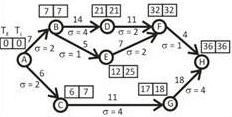So, the critical path is A — B — D — F — H

Critical duration = 36 days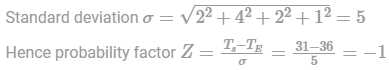Hence probability of completion is 31 days 100 - 84.13 = 15.87%

Practice Test: Civil Engineering (CE)- 15 - Question 49

Calculate the shear strength of a ISWB section of height 350 mm, breadth 200 mm, thickness of web 8 mm.

Detailed Solution for Practice Test: Civil Engineering (CE)- 15 - Question 49

According to LSM,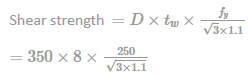= 367.407 kN

Practice Test: Civil Engineering (CE)- 15 - Question 50

An undirected graph C has n nodes. Its adjacency matrix is given by an n × n square matrix whose

(i) diagonal elements are 0’s, and

(ii) non-diagonal elements are l’s.

Which one of the following is TRUE?

Detailed Solution for Practice Test: Civil Engineering (CE)- 15 - Question 50

If all non-diagonal elements are 1, then every vertex is connected to every other vertex in the graph with an edge of weight 1. Such a graph has multiple distinct MSTs with cost n-1. See the below example.

The connected graph: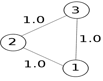Below are three Minimum Spanning trees each of cost 2.0.

Minimum Spanning Tree 1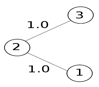Minimum Spanning Tree 2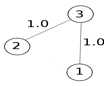Minimum Spanning Tree 3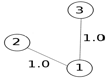Practice Test: Civil Engineering (CE)- 15 - Question 51

The ratio of demand for 2,00,000 persons calculated by Buston’s to Freeman’s formula is

Detailed Solution for Practice Test: Civil Engineering (CE)- 15 - Question 51

Buston’s Formula, Q = 5663√P (P is in thousands) =5663 √200 = 80086.91 lit/min----(i)

Freeman’s Formula, Q = 1136 (P/5 + 10) = 1136(200/5+10) = 56800 lit/min------(ii)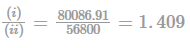Practice Test: Civil Engineering (CE)- 15 - Question 52

In an incompressible flow, the:

Detailed Solution for Practice Test: Civil Engineering (CE)- 15 - Question 52

In an incompressible fluid flow, the density of fluid element will not change during the motion and it serves as the property of fluid flow and not of fluid. Incompressible flow does not imply that the fluid itself is incompressible. Even compressible fluids can give a good approximation – be modelled as an incompressible flow

Thus continuity equation reduces to

∇ · v = 0

Practice Test: Civil Engineering (CE)- 15 - Question 53

Choose the correct statement(s) with reference to determine the denseness of the granular soil.

i. Loosest state is achieved by free fall of about one centimetre

ii. Loosest state is achieved by vibration

iii. Densest state is achieved by static pressure

iv. Densest state is achieved by combination of static pressure and vibration

Detailed Solution for Practice Test: Civil Engineering (CE)- 15 - Question 53

The loosest state for a granular material can usually be created by allowing the dry material to fall into a container from a funnel held in such a way that the free fall is about one centimetre. The densest state can be established by a combination of static pressure and vibration of soil packed in a container.

Practice Test: Civil Engineering (CE)- 15 - Question 54

The equivalent radius resisting section of 20 cm slab, if the ratio of radius of wheel load distribution to thickness of slab is 0.73 is

Detailed Solution for Practice Test: Civil Engineering (CE)- 15 - Question 54

Given thickness of slab (h)=20 cm,

and a/h = 0.73

a = 0.73 × h = 0.73 × 20 = 14.6 cm

As a < 1.724="" />

therefore the equivalent radius of resisting section is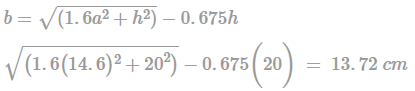Practice Test: Civil Engineering (CE)- 15 - Question 55

A plate load test is conducted using a 600 mm square plate on cohesionless soil. At a load of 0.5N/mm2 applied on the plate, it settles down by 15 mm. Then what can be the minimum size of the square foundation so that the settlement does not exceed the maximum permissible settlement.

Detailed Solution for Practice Test: Civil Engineering (CE)- 15 - Question 55

The maximum permissible settlement on sandy soil is 25 mm.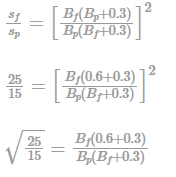1.29 × 0.6(Bf + 0.3) = Bf = 0.2322

0.9Bf = 0.2322

0.126 Bf = 0.2322

Bf = 1.842 ≈ 1.85m.

Practice Test: Civil Engineering (CE)- 15 - Question 56

The following data is about a reservoir near Jiya Sarai, Delhi.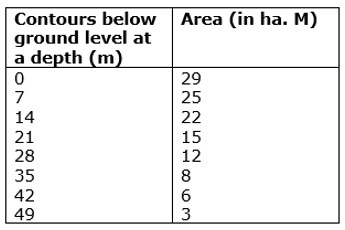Depth of water level from the ground, when the reservoir is 60% full, is _________m.

[Assume volume between two consecutive contours

= (Difference between contours)/2 × (Sum of areas of both contours)

Detailed Solution for Practice Test: Civil Engineering (CE)- 15 - Question 56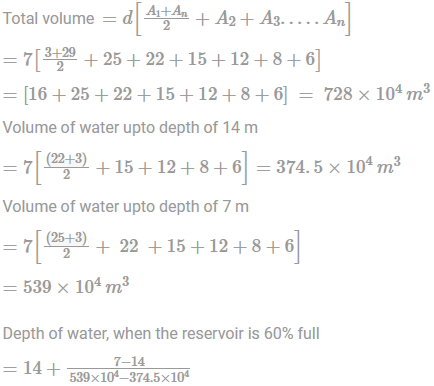(436.8 × 104 - 374.5 × 104)

= 14 - 7/164.5 x 62.3 = 11.3489 m ≃ 11.4 m

Practice Test: Civil Engineering (CE)- 15 - Question 57

Which of the following statements are CORRECT?

1) Measurement of colour is done by colour matching technique, also called Tintometer method.

2) The standard unit of colour is that which is produced by one milligram of platinum cobalt complex dissolved in one litre of distilled water.

3) For public supplies, the colour number on cobalt scale should not exceed 25 and should be preferably less than 5.

Detailed Solution for Practice Test: Civil Engineering (CE)- 15 - Question 57

Measurement of colour is done by colour matching technique, also called Tintometer method.

The standard unit of colour is that which is produced by one milligram of platinum cobalt complex dissolved in one litre of distilled water.

For public supplies, the colour number on cobalt scale should not exceed 25(cause for rejection limit) and should be preferably less than 5(permissible limit).

Hence, all the given statements are correct.

Practice Test: Civil Engineering (CE)- 15 - Question 58

A particle moves along a curve whose parametric equations are : x = t3+2t,y = −3e−2t and z = 2sin⁡(5t), where x,y and z show variations of the distance covered by the particle (in cm) with time t (in s). The magnitude of the acceleration of the particle (in cm/s2) at t = 0 is cm2/s

Detailed Solution for Practice Test: Civil Engineering (CE)- 15 - Question 58

Correct answer is 12 Given equation of motion in 3 different direction

i.e x = t3+2t,y = −3e−2t and z = 2sin⁡5t(cm)

So Velocity in x,y,z directions are Vx = ∂x/∂t = (3t2 + 2)i;Vy = (6e−2t)j;Vz = (10cos⁡5t)kcm/s

Now accelerations in x, y , z directions are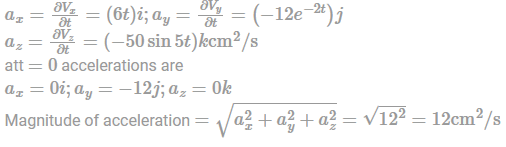Practice Test: Civil Engineering (CE)- 15 - Question 59

For a constant specific energy of 1.5m, the maximum discharge (in m3/s) that may occur in a parabolic channel 5.0 m wide is

Detailed Solution for Practice Test: Civil Engineering (CE)- 15 - Question 59

For a given specific energy, the discharge is maximum when critical flow occurs

Depth of flow, y = yc

y = yc = 3/4E = 3/4 × 1.5 = 1.125 m

For a parabolic channel,

Fr = V√gD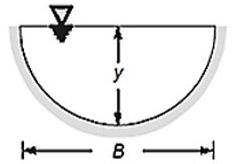A = 2/3 By

T = B

D = A/T = 2/3 y

For critical flow, Fr = 1

∴ V2 = g × 2/3y = 9.81 × 2/3 × 1.125

∴ V = 2.712 m/s

Discharge, Q = AV

= 2/3 × 5 × 1.125 × 2.712

= 10.17 m3/s.Positive_Mark: 2

Practice Test: Civil Engineering (CE)- 15 - Question 60

Two masses are attached to the two ends of a cable which passes over two frictionless pulleys as shown in the figure. (Consider acceleration due to gravity as g). The acceleration of the 100 kg mass is

Detailed Solution for Practice Test: Civil Engineering (CE)- 15 - Question 60

Consider the acceleration 'a' upward for 50kg mass and downward for 100 kg mass.

Now, by applying equation of the equilibrium we have

T-50g = 50a................eq.1

100g-T = 100a.............eq.2

by solving these two equations, we get

a = g/3

Practice Test: Civil Engineering (CE)- 15 - Question 61

Classify the soil with the following properties according to IS classification: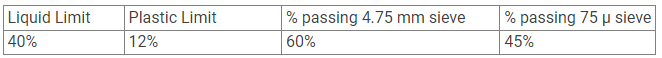Detailed Solution for Practice Test: Civil Engineering (CE)- 15 - Question 61

Fraction upto 4.75 mm = 40%

Fraction between 4.75 mm & 0.075 mm = 15 %

Fraction below 0.075 mm = 45 %

Coarse-grained soils are defined as those in which at least half the material is retained on a 75µm (No.200) sieve.

As more than 50% is retained on 75μ IS sieve, the soil is coarse-grained.

Coarse fraction = 55%

Gravel fraction = 40%

Sand fraction = 15%

As more than half the coarse fraction is larger than 4.75 mm sieve, the coarse part contains mainly gravel(G).

Now for classification under fines use A-Line concept

Given, LL = 40% & PL = 12%

IP = 40-12 = 28 % and from A-Line equation,

IP = 0.73(LL-20) = 14.6%

Hence, point is above A-Line = > Clay(C)

=> GC

Practice Test: Civil Engineering (CE)- 15 - Question 62

The magnetic bearing of a line was N 60 °30' W when the declination was 5 °E. The magnetic bearing of line, if declination has changed to 3 °W, is

Detailed Solution for Practice Test: Civil Engineering (CE)- 15 - Question 62

Initial magnetic bearing = N 60 °30' W

= 360 - 60 °30' = 299 °30'

Initial declination = 5 °E

∴ True bearing = Magnetic bearing + East declination

= 304 °30'

Present declination = 3 ° W

True bearing always remains same

∴ Present magnetic bearing = True bearing + West declination

= 307 ° 30'

= N 52 °30'W

Practice Test: Civil Engineering (CE)- 15 - Question 63

Two dimensional stress tensor at a point is given by a matrix

[σxx τxy τyx σyy] = [100 30 30 20 ]MPa

The maximum shear stress is _______ MPa.

Detailed Solution for Practice Test: Civil Engineering (CE)- 15 - Question 63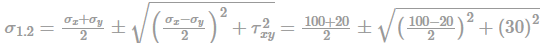= 60 ± 50 σmax = 60 + 50 = 110

σmin = 60 - 50 = 10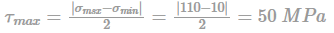Practice Test: Civil Engineering (CE)- 15 - Question 64

For the given section of RCC beam, the neutral axis depth by working stress method is _________mm.

[Take M20 grade of concrete and Fe415 steel]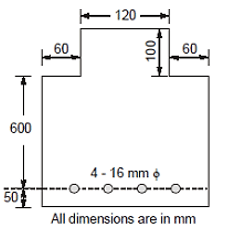Detailed Solution for Practice Test: Civil Engineering (CE)- 15 - Question 64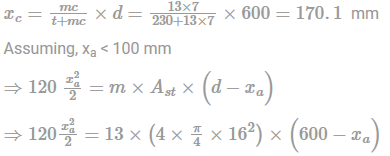xa = 247>100 mm

Assumption is wrong.

Now consider xa < 100="" />

Equating the moment of area

120 × 100 × (xa-50) + 240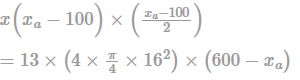∴ xa = 223.96 mm

Practice Test: Civil Engineering (CE)- 15 - Question 65

Available chlorine in bleaching powder solution is 0.6 mg/ml. To obtain a chlorine dose of 0.1 mg/lit in a 200 ml water sample, the amount of bleaching powder solution to be added is

Detailed Solution for Practice Test: Civil Engineering (CE)- 15 - Question 65

The amount of chlorine needed in 400 ml water sample = (0.1/1000 × 200) mg = 0.02 mg----(i)

Let x ml be the amount of bleaching powder solution

The amount of chlorine present in x ml of bleaching powder solution = x × 0.6 mg----(ii)

Thus, (0.02) = x × 0.6 ⇒ x = 33.33 × 10-3 ml.

Information about Practice Test: Civil Engineering (CE)- 15 Page
In this test you can find the Exam questions for Practice Test: Civil Engineering (CE)- 15 solved & explained in the simplest way possible. Besides giving Questions and answers for Practice Test: Civil Engineering (CE)- 15, EduRev gives you an ample number of Online tests for practice(Scan QR code)